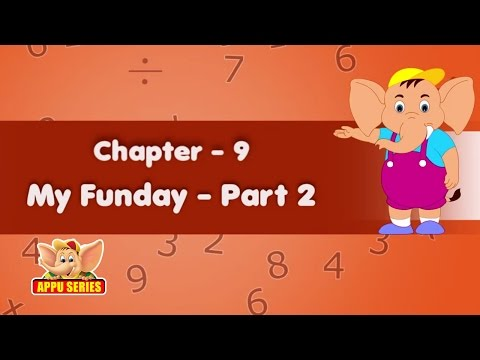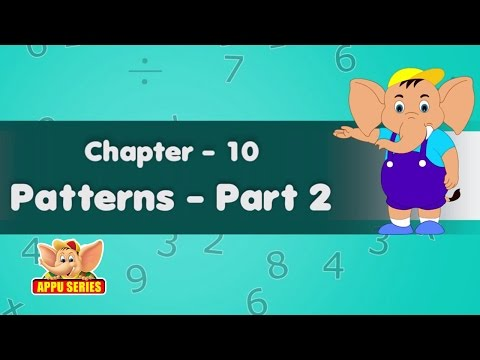Courses

# Patterns - 2

## 20 Questions MCQ Test Science Olympiad Class 4 | Patterns - 2

Description
This mock test of Patterns - 2 for Class 4 helps you for every Class 4 entrance exam. This contains 20 Multiple Choice Questions for Class 4 Patterns - 2 (mcq) to study with solutions a complete question bank. The solved questions answers in this Patterns - 2 quiz give you a good mix of easy questions and tough questions. Class 4 students definitely take this Patterns - 2 exercise for a better result in the exam. You can find other Patterns - 2 extra questions, long questions & short questions for Class 4 on EduRev as well by searching above.
QUESTION: 1

### Find the letter which will end the first word and start the second word.​ SAL?ILL

Solution:

The answer is T because it completes the first word SALT and also begins the second word TILL.

QUESTION: 2

Solution:
QUESTION: 3

### Find the letter which will end the first word and start the second word.​ DUC?ITE

Solution:

The answer is E as it completes the first word; DUCK and also begins the second word KITE.

QUESTION: 4

Find the letter which will end the first word and start the second word.​
MAT?VER

Solution:
QUESTION: 5

Find the letter which will end the first word and start the second word.​
RUI?EAR

Solution:

The answer is N as it completes the first word; RUIN and also begins the second word NEAR.

QUESTION: 6

In each of the following questions which alternative will replace the question mark.
​FB is to GD as PM is to

Solution:

F + 1 = G; B + 2 = D. Similarly, P + 1 = Q, M + 2 = O.

QUESTION: 7

In each of the following questions which alternative will replace the question mark.
​NA is to LF as XN is to

Solution:

N – 2 = L; A + 5 = F. Similarly, X – 2  = V, N + 5 = S.

QUESTION: 8

In each of the following questions which alternative will replace the question mark.
FH is to DF as UY is to

Solution:

F – 2 = D; H – 2 = F. Similarly, U – 2  = S, Y – 2 = W.

QUESTION: 9

In each of the following questions which alternative will replace the question mark.
​KM is to OJ as VH is to

Solution:
QUESTION: 10

In each of the following questions which alternative will replace the question mark.
DI is to EE as RQ is to

Solution:

D + 1 = E; I - 4 = E. Similarly, R + 1 = S, Q - 4 = M.

QUESTION: 11

In each of the following questions which alternative will replace the question mark.

GH is to KL as PQ is to

Solution:
QUESTION: 12

In each of the following questions which alternative will replace the question mark.

CK is to KS as MU is to

Solution:
QUESTION: 13

In each of the following questions which alternative will replace the question mark.
YW is to VT as NL is to

Solution:
QUESTION: 14

In each of the following questions which alternative will replace the question mark.
DH is to KO as RV is to

Solution:
QUESTION: 15

In each of the following questions which alternative will replace the question mark.
FG is to JK as NO is to

Solution:

F + 4 = J; G +4 = K. Similarly, N + 4 = R, O + 4 = S.

QUESTION: 16

In each of the following questions which alternative will replace the question mark.
JK is to NA as QH is to

Solution:
QUESTION: 17

In each of the following questions which alternative will replace the question mark.
NT is to HP as WE is to

Solution:
QUESTION: 18

In each of the following questions which alternative will replace the question mark.
XL is to XF as YP is to

Solution:
QUESTION: 19

In each of the following questions which alternative will replace the question mark.

4 is to 16 as 16 is to

Solution:
QUESTION: 20

In each of the following questions which alternative will replace the question mark.
3 is to 12 as 12 is to

Solution:

Track your progress, build streaks, highlight & save important lessons and more!

### Similar Content### Related tests